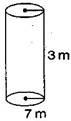# A closed cylindrical tank of radius 7 m and height 3 m is made from a sheet of metal. How much sheet of metal is required?Given

The radius of a cylindrical tank, r = 7 m

Height of cylindrical tank , h = 3 m

Find out

We have to find out the amount of metal sheets required

Solution

Total surface area of cylindrical tank = 2πr(h+r)

= 2×(22/7)×7(3+7)

= 44×10 = 440

Therefore, 440 m2 metal sheet is required.